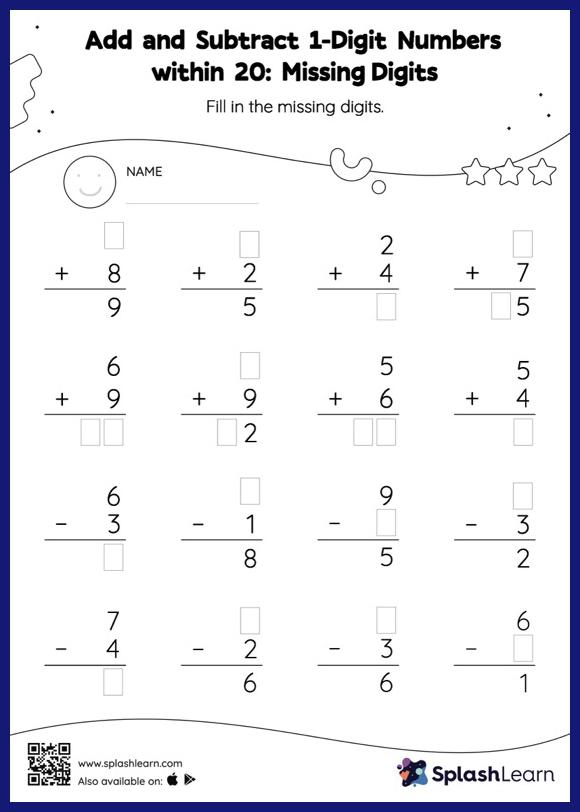# Add and Subtract 1-Digit Numbers within 20: Missing Digits Worksheet

Home > Add and Subtract 1-Digit Numbers within 20: Missing DigitsThis worksheet invites students to add and subtract 1-digit numbers within 20, which helps them become proficient in addition and subtraction. In add and subtract 1-digit numbers within 20 worksheet, students must find the missing number using the count-on or count back method or the relationship between addition and subtraction. This worksheet is about practicing the column method in which numbers are written one on top of another. In this method, students use their place value understanding to solve the problems.